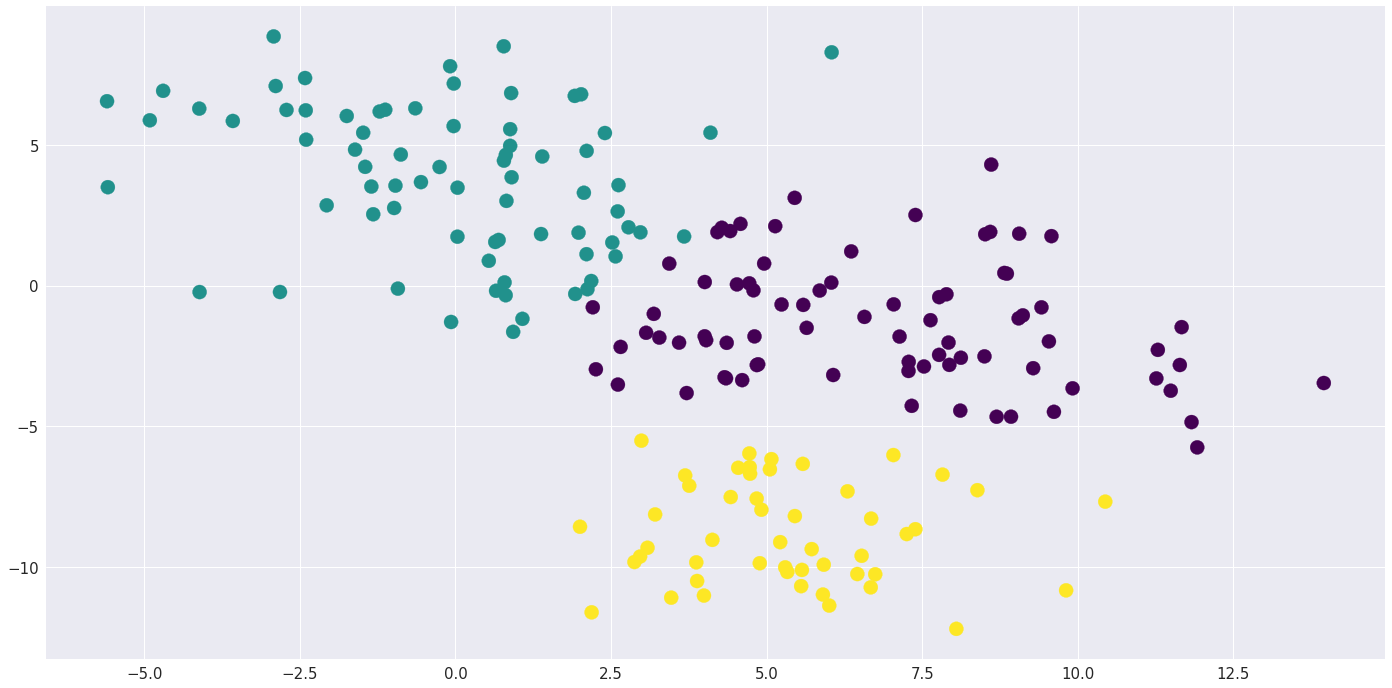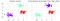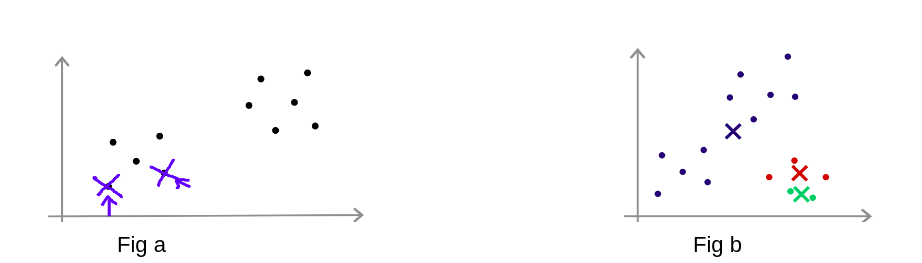# (Bottom’s Up Approach)Clusters found by KMeans Algorithm;

Suppose you are a data scientist at a clothing company and you have a data set with features height and weight and you want to find clusters(i.e groups of Small, Medium, Large fitting of clothes) so that your company can make clothes accordingly and give your customers a satisfaction with awesome fitting by your brand and TBH you might get a raise for that 😛

KMeans is an unsupervised learning algorithm. K-means clustering is a classification algorithm used to automatically divide a large group into smaller groups.

This comes under unsupervised learning since you don’t have the labels here just height and weight ratio. But, What the hack is KMeans?

As far you know that it is a clustering algorithm,
Wait, see this

You human, can you find three clusters over here? Awesome!

Our machine can also find the clusters and KMeans is one of the algorithm in finding clusters. Machine can’t find on its own we have to teach it!

So, what KMeans does is it find the clusters (best way to find the number of clusters is by manually specifying how many clusters are in the data, or by elbow method. Excited? Just 4 mins more and I’ll make it worth!

This is the code for finding clusters in the data using sklearn.cluster.KMeans

On running this code we’ll get this…LHS clusters are formed via KMeans labels and RHS clusters are made from the labels that we knew already!

What’s going on under the hood?

But you know what, Only 3 steps are there in this algorithm. Only 3!

## Step 0: Randomly initialize 3 points because we know there are 3 clusters!

(If we don’t know clusters, we can find by elbow method, or by experimenting, visualizing manually)

## Step 1: Cluster Assignment Step

In this step, we will go through each example(training points) and on whichever cluster it is closest to, that particular cluster is assigned to that training example.
This means to go through the dataset and color each point depending on the color of the centroid.

## Step 2: Move Centroid Step

After the cluster assignment step, we’re going to move the centroid to the average point of the cluster and update the centroid. This is the new centroid (in simple terms, look at the cluster and it’s colored dots and computes their(dots) mean and move centroid at that position.

This is repeated until this algorithm converges. Converges means that after repeating the algorithm, centroid and it’s points doesn’t change significantly!

This can be done easily by python’s sklearn.clusterKMeans, But here we gonna understand what’s going on under the hood!

The first image that you saw was also plotted via clusters formed by KMeans.

This was just a theory, want to dive little bit deeper with me? C’mon, this gonna be fun!

# Steps, in depth

For cluster assignment step we minimize the cost function i.e

Let each example(training points) be x¹, x², x³,…,x^m and μ¹, μ²,…,μ^k represent the cluster’s centroid number and k be the number of clusters.

For each assignment step, we minimize cost function so that the distance between cluster’s centroid and the training example be minimum and in whichever cluster’s centroid cost turns out to be minimum that data point is assigned to that particular cluster.

What the hack is cost function now?
Cost function is the square error of the distance b/w the data point and the centroid, we minimize this error,
Cost Function(J) = 1/m(Σ||xi - μk||²)
Why minimizing the square? Good question, actually it is just a convention! :p

c:= min ||xi - μk||²
(Please, note. The value of k that minimizes the cost function is assigned to c)

We are minimizing the mean square distance b/w the data point and cluster’s centroid

## Algorithm, The heart!

Repeat until algo converges{
# m is the number of examples; k is the number of centroid; xi is data point; ci is the value of K(cluster) that minimizes the cost!

For i:= 1 to m

ci := index(from 1 to K) of cluster centroid, closest to xi

For k=1 to K

μk := average of points assigned to cluster k

}

Cluster assignment step is minimizing the J(cost function) wrt c¹, c²,… holding μ¹, μ²,..;
Move cluster step chooses the value of μ that minimizes J(Cost Function)

# A Big Problem with KMeans

We know that we initialize cluster centroid randomly but it is possible that KMeans can end up converging to different solutions depending on how the clusters are initialized.
If we have unlucky random initialization we might stuck at local optimaIn both of the above figures these correspond to bad local optima, because in (fig a) we can’t do a shit to change the clusters and in (fig b) it has taken cluster 1 and 2 and used them in one cluster and also it has poorly split third cluster i.e red and green one’s

So, To increase the odds in finding the best possible clustering we need to do multiple random initialization, i.e initialize KMeans alot of time and pick clustering that gave the lowest cost J.

All these steps are taken by sklearn.cluster.KMeans algorithm under the hood!

If you’d like to see this in action, visit this awesome site by Stanford

Questions? Comments? Let’s connect on Twitter! or
drop a mail at adityakaushik471@gmail.com

I strive to make computers learn! ;)

## More from Aditya Kaushik

I strive to make computers learn! ;)